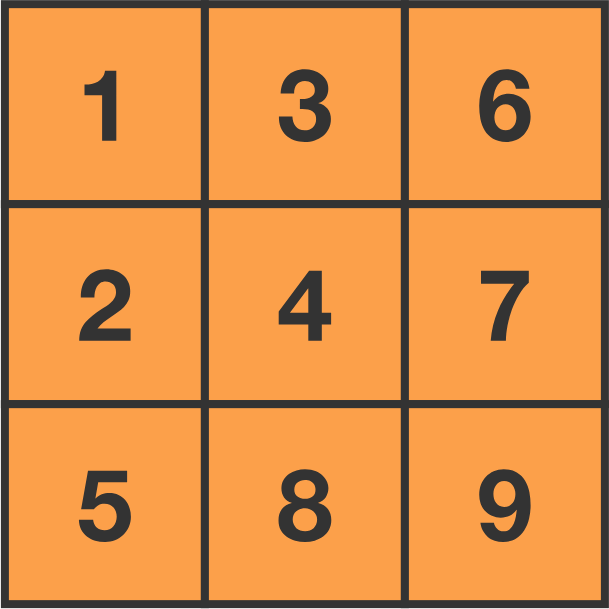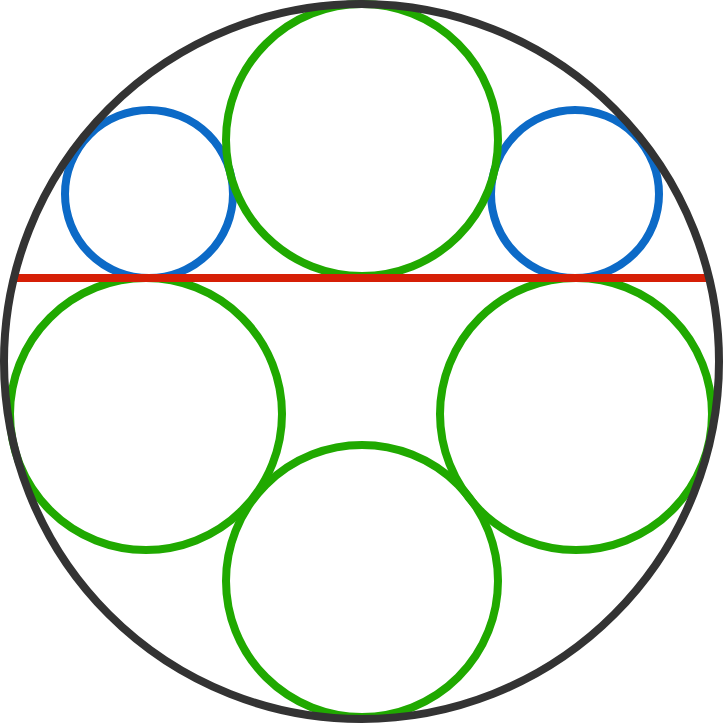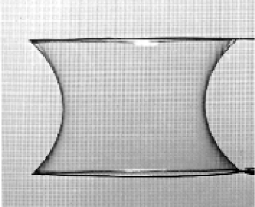# Problems of the Week

Contribute a problem

# 2018-09-17 Advanced

The first few terms of the Fibonacci sequence are as follows: \begin{aligned} F_1 = 1,&\, F_2 = 1,\, F_3 = 2,\, F_4 = 3,\,\\\\ {\color{#D61F06}F_5 = 5},&\, F_6 = 8,\, {\color{#D61F06}F_ 7 = 13},\, F_8 = 21, {\color{#D61F06}F_9 = 34},\, … \end{aligned} It just so happens that $5$, $13$, and $34$ are also the hypotenuses of right triangles with all integer sides: $(3, 4, {\color{#D61F06}5}),\ (5, 12, {\color{#D61F06}13}),\ (16, 30, {\color{#D61F06}34}).$ Is every Fibonacci number $F_{2n - 1}$ for all $n \geq 3$ the hypotenuse of a right triangle with integer sides?How many ways can you arrange the numbers 1 through 9 in a $3 \times 3$ grid such that the following conditions hold?

• Every number is greater than the number directly above it.
• Every number is greater than the number immediately to the left of it.

Inspired by this problem.

\begin{aligned} S&=\sqrt{x+2}+\sqrt{y+5}+\sqrt{z+10}\\\\ T&=\sqrt{x+1}+\sqrt{y+1}+\sqrt{z+1} \end{aligned}

If the above is true for $x, y, z >0,$ what is the minimum value of $S^{2}-T^{2}?$Four congruent green circles and two congruent blue circles are tangential to one another, to the red chord, and to the large black circle, as shown.

If $r_1$ is the radius of the green circles and $r_2$ is the radius of the blue circles, find the ratio $\frac{r_1}{r_2},$ which can be expressed as $\frac{a+b\sqrt{d}} {c}$, where $a, b, c$ are coprime integers and $d$ is a square-free integer.

Give the answer as the product $a \times b \times c \times d$.A soap bubble stretches between two identical unit rings (with radius 1).

What is the maximum separation between the two rings before the bubble pops?

×

Problem Loading...

Note Loading...

Set Loading...